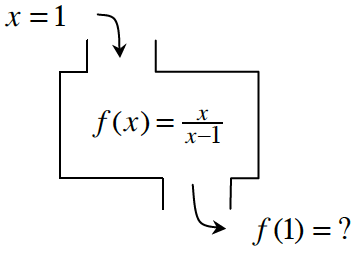### Home > GB8I > Chapter 2 Unit 2 > Lesson INT1: 2.1.2 > Problem2-21

2-21.

Calculate the output for the function with the given input. If there is no possible output for the given input, explain why not.

Substitute $1$ in for $x$ and evaluate the function.

$f(1)=\frac{1}{1-1}$

$f(1)=\frac{1}{0}$

What does it mean when there is a zero in the denominator?